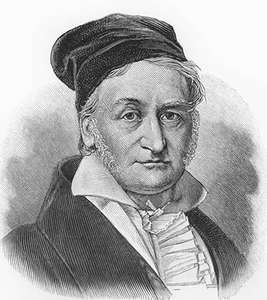# My favourite mathematicianThe name of my favourite mathematician is Friedrich Gauss ,He was a german scientist and a mathematician who has made significant contributions to many fields in mathematics .He was sometimes referred to us the 'princeps mathematicorum ' meaning the foremost mathematician antique .His exceptional influence in mathematics has ranked him most influential mathematician in history.
https://en.m.wikipedia.org/wiki/carl friedrich Gausssome of his contributions in mathematics are as follows

Geodesy
He founded geodesy which is the science that measures accuracy and understanding of the our planet's geometric shape and its orientation in space and its gravity.Geodesy is so important that it has an entire program dedicated to geodetic informationFunction theory refers to theory of functions of a real variable, the traditional name of real analysis, a branch of mathematical analysis dealing with the real numbers and real-valued functions of a real variable and geometric function theory, the study of geometric properties of analyticGeometry deals with the arithmetic, one of the oldest branches of mathematics. It is concerned with properties of space that are related with distance, shape, size, and relative position of figures .mathematician who works in the field of geometry is called a geometer..
geometry,which includes the notions of point, line, plane, distance, angle, surface, and curve, as fundamental concepts.https://en.m.wikipedia.org/wiki/Geometry
Probability theory
Probability theory, a branch of mathematics concerned with the analysis of random phenomena. The outcome of a random event cannot be determined before it occurs, but it may be any one of several possible outcomes. The actual outcome is considered to be determined by chance.https://en.m.wikipedia.org/wiki/probability